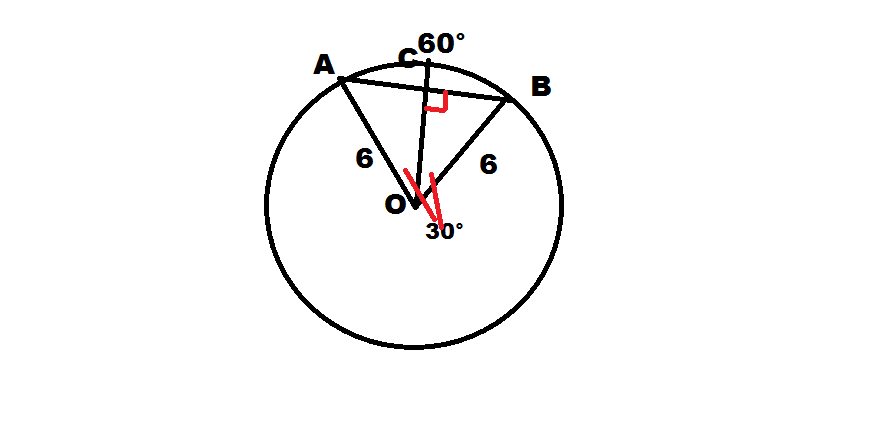## All You Should Know About the Chord of a CircleThe chord of a circle can be perfectly defined as the line segment that helps in joining any two points on the circumference of the circle and further it is very much important to be noted for the students that diameter is considered to be the longest chord in any of the circle. This particular chord always passes through the centre of the circle and it is very much important for the students to be aware of this particular concept so that they can learn the other aspects associated with the circle very easily and perfectly. There are two formulas to be learnt by the students in this particular area to find out the length of the chord.

#### Those formulas are explained as follows:

The length of the chord using the perpendicular distance from the center can be found with the utilisation of the following formula:

Cord length is equal to 2 into under root of radius square minus perpendicular distance from the chord to the center square

The second formula can be found out from the world of trigonometry and it can be implemented in the following manner:

The chord of the circle will be equal to 2 into radius into sin(c/2), C here means the angle which is subtended at the center by the chord

There are different kinds of theorems available in this particular area as well which the students need to be aware of and some of those theorems along with the main statements are mentioned as follows:

• Equal chord equal angles theorem: As per this particular theorem the chords will be equal in length which will be subtending the equal angles at the center of the circle. The conversion of this particular theorem is also very much true and if two angles suspended by two chords at the center are equal then the chords will also be of equal length.
• An equal angle equal chord theorem conversion of theorem one: As per this particular theorem the angle subtended by the chords of a particular circle will be equal in measure then the length of the chord will also be equal.
• Equal chords will be equidistant from the center theorem: As per the statement of this particular theorem the equal chords of the circle will be equidistant from the center of the circle and this is a very relevant theorem in the world of mathematics.

Many people are confused about the concept of diameter as a chord of the circle but it is to be remembered that diameter is the longest chord which is possible into a particular circle and it will help in dividing the circle into two equal parts. So, it is to be remembered by the students that the diameter of circle will also be a chord and there is no need to get confused between the two of these terms. These are the terms with the same meaning and it is further very much important for the people to remember different kinds of concepts associated with the circle for example circumference of the circle, the radius of the circle, properties of the circle, and several other kinds of points.

#### Some of the Most Common Properties of the Circle are Mentioned:

1. The circles are said to be congruent if they will have the equal radius
2. The diameter is the longest chord of the circle
3. The equal chord in the equal circle will have an equal circumference
4. The radius which is drawn perpendicular to the chord will help in bisecting the chord

There are several other kinds of properties as well but all the above-mentioned properties are directly linked with the concept of the chord which is the main reason that students must be made of it. Apart from this, it is the responsibility of the students to get them enrolled at professional platforms like Cuemath’s website so that they can become experts in the field without any kind of hassle.

## 7 Genuine Reasons To Invest In Travel Insurance

Life is quite unpredictable and worse things like accident might occur just about anywhere. But the risks become manifolds if you are travelling abroad to some new country. Foreign countries do not follow your country’s rules & regulations. Hence, if anything happens when overseas, then it can be a major […]